Function Repository Resource:

# BitTest

Determine whether a given bit in an integer is set to one

Contributed by: Daniel Sanchez
 ResourceFunction["BitTest"][n, k] returns True if the kth (starting from zero) bit of n is set to 1 and returns False otherwise. ResourceFunction["BitTest"][n, {k1,k2, …}] returns a list of truth values for each ki. ResourceFunction["BitTest"][n, {k1,k2, …}, op] applies the logical operator op to the list of truth values.

## Details and Options

ResourceFunction["BitTest"][{n1,n2, }, k] tests if the bit at position k is set for each ni.
ResourceFunction["BitTest"][n, k] is equivalent to BitAnd[n, 2k] > 0.
ResourceFunction["BitTest"][n, {k1,k2, }] is equivalent to ResourceFunction["BitTest"][n, {k1,k2, }, List].
ResourceFunction["BitTest"][n, {k1,k2, }, op] is equivalent to Apply[op][ResourceFunction["BitTest"][n, ki]].
The logical operators supported are And, Nand, Nor, Or and Xor.

## Examples

### Basic Examples (2)

Display coefficients at powers of 2 in the integer 42:

 In:=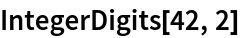Out=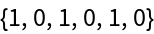Determine whether the bit at position 3 is set:

 In:=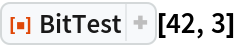Out=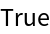Test whether bits 0 through 5 are set:

 In:=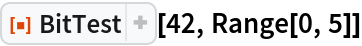Out=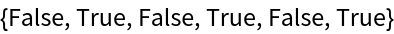Various logical operators can be passed down as a third argument:

 In:=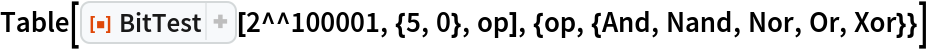Out=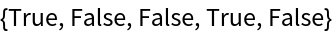### Applications (1)

Test whether a 4-bit register should set a carry flag after performing addition:

 In:=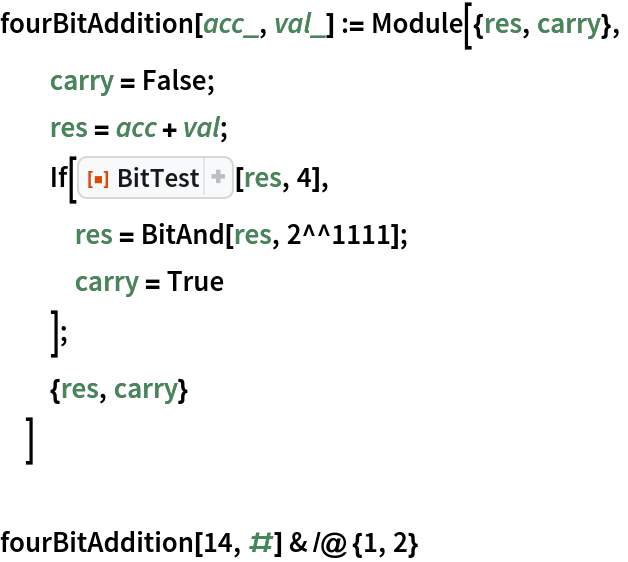Out=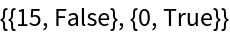### Properties and Relations (2)

The following are equivalent:

 In:=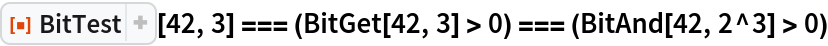Out=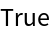The DataStructure "BitVector" has this function as one of its methods:

 In:=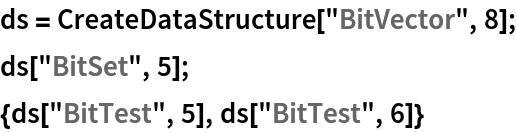Out=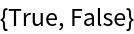Daniel Sanchez

## Version History

• 1.0.0 – 10 November 2020